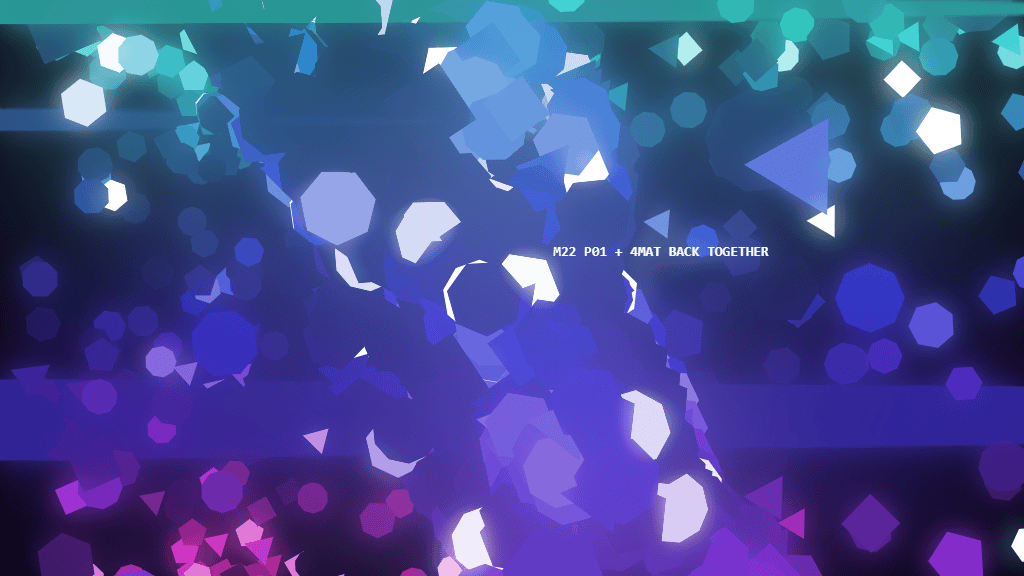# M22

535 words ~ 3-5 minsMathieu 'p01' Henri on August 6th, 2022

# M22

M22: DEMO-lishing 1kb competition since 2012 using Javascript and the web platformThe Assembly is a demoscene event with the most anticipated 1kb, 4kb and full size demo competitions. I am absolutely thrilled to be part of the competition again.

## DEMO-lishing 1kb competition since 2012

M22 celebrates the 10 years anniversary of MATRAKA, my first heavy 1kb intro that won the DEMOJS 1kb competition and set JavaScript 1kb to a new level.

M22 pushes everything I've done in 1kb to even further with more advanced music, mixing, timeline, compression technique, ...

## HD video capture with original voices

Here's an HD video capture with original voices to watch M22 in the best possible way in case your current web browser or set up isn't suitable

## Technical break down

It is still very early after the release, and I promise to go into much more details in the comming days... but I can assure you it's been a blast and absolutely brutal to get this all in 1024 bytes in 3 weeks with a new/old workflow.

### Week by week break down

The first week was about getting the basic setup; the render loop, the main shape, camera and most basic audio.

The second week was looking other compression techniques than the PNG+HTML polyglot approach I used before.

The last week was about bringing M22 to 11; working on the direction, music (adding more channels and instruments, + some reverb on the lead insrument), mixing, timeline, speech synth + text render, ... not thinking about the 1024 bytes limit.

In the last 3 days, were all about improving and optimizing the code. M22 went through 5974 iterations slowly going from 1521 to 1024 bytes compressed with Brotli-11.

That's 5974 individual changes made, compressed and tested.

## Source code

The entire source code is 1943 bytes long and compresses down to 1024 bytes using Brotli-11

``````Click<canvas id=c><script>onclick=(e,p)=>{c.style="position:fixed;width:100%;height:100%;background:#000";g=[];
w=Math.PI;s=Math.sin;A=new AudioContext;a=A.createScriptProcessor(2048,c.style.top=c.style.left=t=M=l= 0,1);a.connect(A.destination);a.onaudioprocess=(e,p)=>{c.width=1024;c.height=576;j=t>>5;m=s(Math.min(1,t/160)*w)**.5;d=e.outputBuffer.getChannelData(e=t/32%1);o=["","M22\nP01 + 4MAT BACK TOGETHER","","M22\nP01 + 4MAT ROCKING THE 1K AGAIN","","1K COMPETITION OVER"];
if(j>M)a=new SpeechSynthesisUtterance(o[j]),a.lang="en",speechSynthesis.speak(a),M+=1;
f=1-e
h=e**16/16+f**64
l+=[e/8,1,h-.5,1,h-.5,1][j]/64
for(a=0;a<2048;a++){p=g[a]||a
u=3+((p.a/4+4*l)&7)
z=p.z*8;
b=c.getContext("2d");
b.beginPath();
b.fillStyle=p.f;
for(i=0;i<u;i++){
v=i/u*w*2-4*l-p.x/2048;
b.lineTo(p.x+z*s(v),p.y+z*s(v+11));
}
if(2<z)b.fill();
d[a]=m*(s(Math.tan(t*l))/64+Math.random()*h+"80411"[j]*(":IW7%,A".charCodeAt(a%7)*t%.1)/8+"13107103135701314204"[(t/4&4)+(t*8&15)]*64*t%1*[e**16/16,.5,h/2,.5,f**16/2,t-=.5][j]+"13107103135701314204"[(t/4&4)+(t*8&15)]*32*t%1*[e**16/16,.5,h/2,.5,f**16/2,t+=.5][j]/2+"12020"[j]*(t*8&7^5?0:Math.random()/8))
x=s(a**2)*256;
y=s(a**3)*128+s(x+l)*8;
r=s(x)*256;
y/=m;
u=(a+x%1)/22%1;
v=(a+y%1)/968;
if(v<1){
y=256;i=32+s(u*w*2*2-4*l)*s(v*w*2*3+l)*16;r=i*s(v*w*2+11);
if(j>0)y=128-u*256,x=i*s(v*w*2)+y*s(l+11)/2
if(j>2)x=i*s(u*w+11),y=i*s(v*w*2)*s(u*w),r*=s(u*w)
if(j%2<1)x/=f**16,y/=f**16,r/=f**16
}z=1024/(256-r*s(l+11)+x*s(l));t+=1/A.sampleRate
g[a]=a?{z,a,x:512+z*(x*s(l+11)+r*s(l)),y:288+z*(y+s(l*8)*16),f:"hsl("+[y/2+32*t,[0,64,0,64,64,64][j]+"%",(j%2*32*m+32*s(u*w*2*2-4*l)*s(v*w*2*3+l+11)+((a%27^a/27-l)%15||64))+"%"]}:{f:"#000",x:512,y:288,z:t*2-256}}
g.sort((e,p)=>e.z-p.z)
b.fillStyle="#fff"
b.font="900 14px monospace";
b.fillText(o[j].slice(0,s(e*w)*78),512+e*64,256)
b.globalCompositeOperation="lighten"
if(j>0)b.drawImage(c,0,0,1024*128,576)
b.filter="blur(1em"
b.drawImage(c,0,0)
b.filter="blur(7em"
b.drawImage(c,0,0)
}
}
</script>``````

That's it.

You see, no external libraries or resources.

Copy and paste this into a new file or even some web playground if you want to see for yourself.